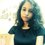# Squaring of Integers in 2 easy steps!

I just figured out this amazing way of squaring numbers with ones digit 5. Suppose you need to find out the square of 35. Then simply square 5 and write down 25 at last and then multiply 3*3 and add 3.That is square the remaining numbers except 5 and add the same value.You will get 12.Since you can also write it as 3 (3) + 3 = 3 (1 + 3) = 3 *4. So,you may just as well multiply the next integer. You can now easily write 35^2 = 1225. In case of another no.,say 125,you take 25 at last and multiply 12 * 13.So,you have 15625. If you are more comfortable in squaring,simply square 12,you'll get 144 and then add 12,you will get 156.So,125^2 = 15625. Rule works for no. whose ones digit is 5. You will probably not find this anywhere else.It was something I came up with while randomly squaring integers.Note by Titas Biswas
6 years, 5 months ago

This discussion board is a place to discuss our Daily Challenges and the math and science related to those challenges. Explanations are more than just a solution — they should explain the steps and thinking strategies that you used to obtain the solution. Comments should further the discussion of math and science.

When posting on Brilliant:

• Use the emojis to react to an explanation, whether you're congratulating a job well done , or just really confused .
• Ask specific questions about the challenge or the steps in somebody's explanation. Well-posed questions can add a lot to the discussion, but posting "I don't understand!" doesn't help anyone.
• Try to contribute something new to the discussion, whether it is an extension, generalization or other idea related to the challenge.
• Stay on topic — we're all here to learn more about math and science, not to hear about your favorite get-rich-quick scheme or current world events.

MarkdownAppears as
*italics* or _italics_ italics
**bold** or __bold__ bold
- bulleted- list
• bulleted
• list
1. numbered2. list
1. numbered
2. list
Note: you must add a full line of space before and after lists for them to show up correctly
paragraph 1paragraph 2

paragraph 1

paragraph 2

[example link](https://brilliant.org)example link
> This is a quote
This is a quote
    # I indented these lines
# 4 spaces, and now they show
# up as a code block.

print "hello world"
# I indented these lines
# 4 spaces, and now they show
# up as a code block.

print "hello world"
MathAppears as
Remember to wrap math in $$ ... $$ or $ ... $ to ensure proper formatting.
2 \times 3 $2 \times 3$
2^{34} $2^{34}$
a_{i-1} $a_{i-1}$
\frac{2}{3} $\frac{2}{3}$
\sqrt{2} $\sqrt{2}$
\sum_{i=1}^3 $\sum_{i=1}^3$
\sin \theta $\sin \theta$
\boxed{123} $\boxed{123}$

## Comments

Sort by:

Top Newest

Very good that you $\color{#D61F06}{rediscovered}$ this yourself. You may be interested in knowing that square of any two and three digit numbers can be found as under. $(10a\pm b)^2$ write square of a and then of b. (if b is 1, 2 , or 3, write its square as 01, 04 or09) to this $\pm$ 20 times a*b. Say $37^2 = (30+7)^2=\color{#D61F06}{9} \color{#3D99F6}{49} +20*21 = 1369. \\37^2 = (40-3)^2=\color{#D61F06}{16} \color{#3D99F6}{09} -20*12=1369 ~\\ Three ~digit~ number ~the ~same ~way~~\\127^2 =(120+7)^2= \color{#D61F06}{144} \color{#3D99F6}{49} +20*84=16129 \\127^2 = (130-3)^2=\color{#D61F06}{169} \color{#3D99F6}{09} -20*39=16129\\With~5~at~ unit ~place ~it ~is ~ easy.~~~ (10a +5)^2= \color{#D61F06}{a*(a+1)} \color{#3D99F6}{25}.\\125^2=(120 +5)^2= \color{#D61F06}{(12*13)} \color{#3D99F6}{25}= \color{#D61F06}{156} \color{#3D99F6}{25} .$

- 6 years, 5 months ago

Log in to reply

Amazing! Thank you.

- 6 years, 5 months ago

Log in to reply

I have made an addition.

- 6 years, 5 months ago

Log in to reply

Thanks again.Learnt 2 more ways at a go.

- 6 years, 5 months ago

Log in to reply

×

Problem Loading...

Note Loading...

Set Loading...